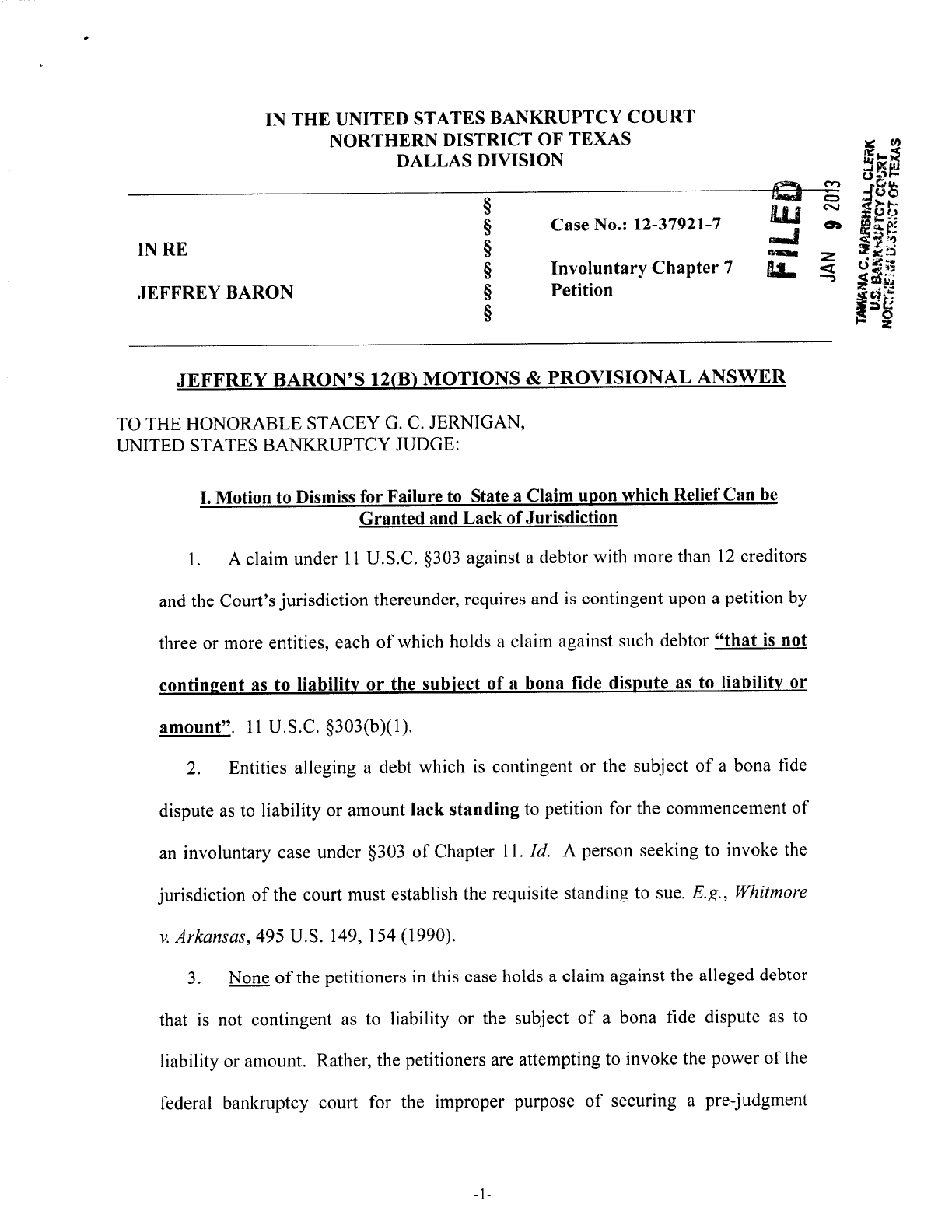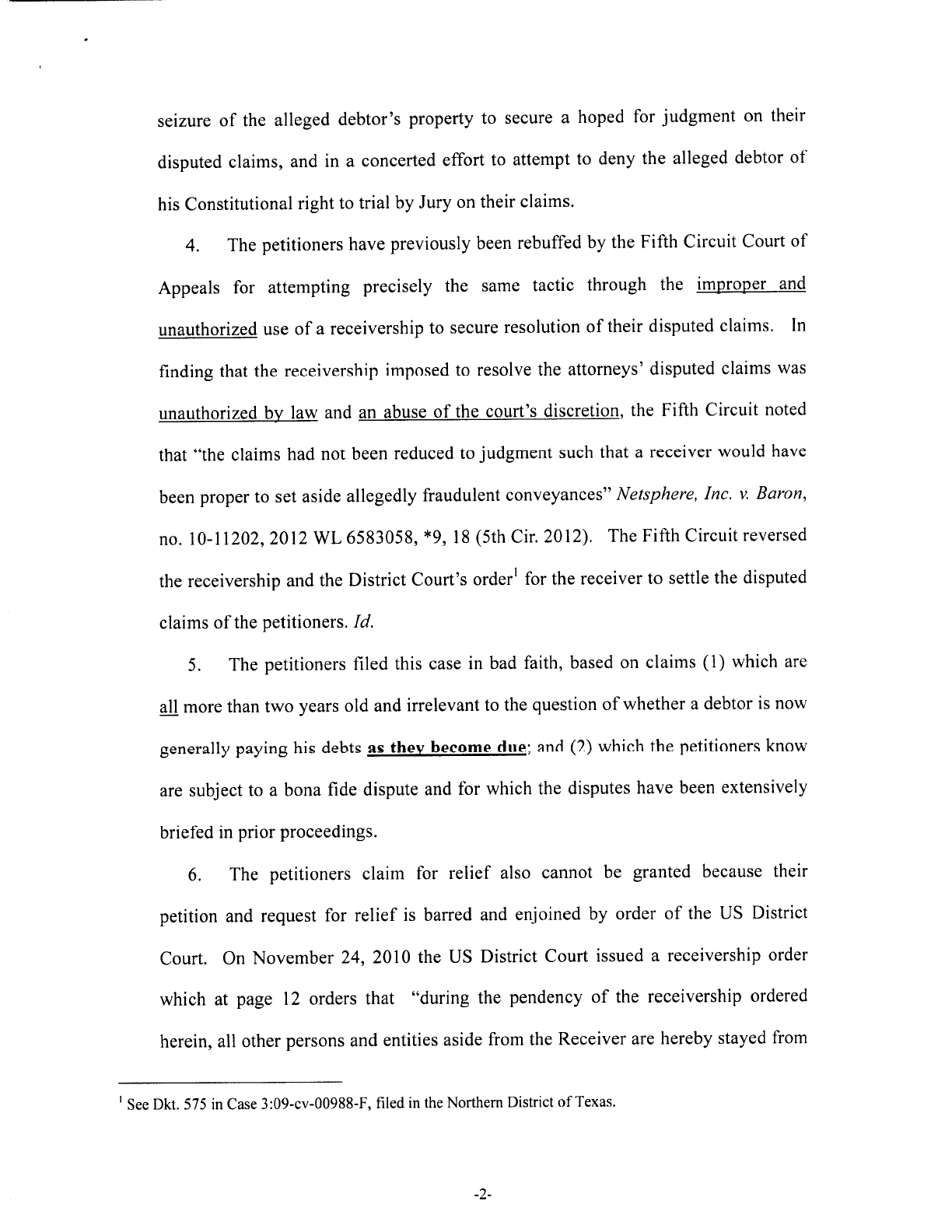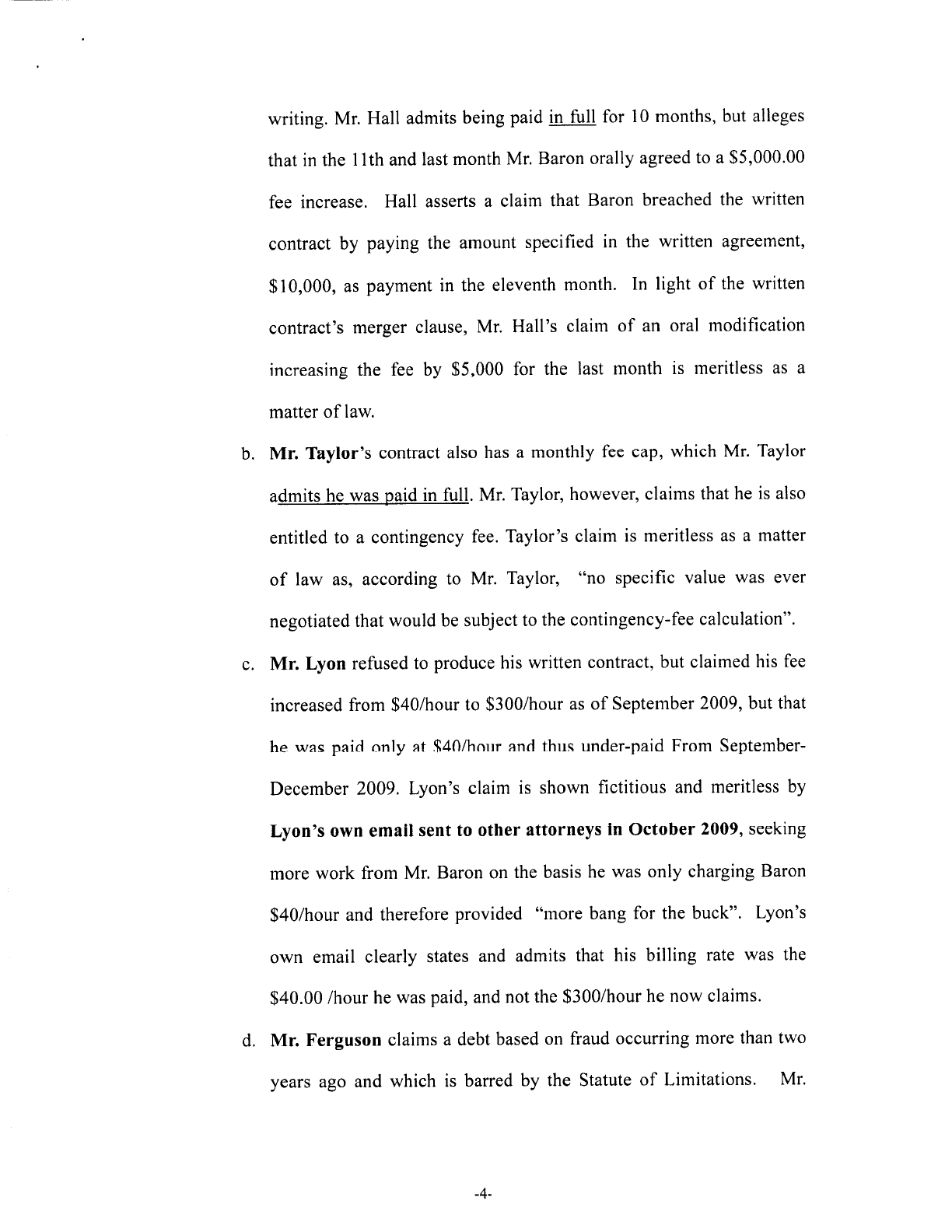```

/*!
* Base CSS for pdf2htmlEX
* Copyright 2012,2013 Lu Wang <coolwanglu@gmail.com>

/*!
* Fancy styles for pdf2htmlEX
* Copyright 2012,2013 Lu Wang <coolwanglu@gmail.com>

.ff0{font-family:sans-serif;visibility:hidden;}
.m0{transform:matrix(0.375000,0.000000,0.000000,0.375000,0,0);-ms-transform:matrix(0.375000,0.000000,0.000000,0.375000,0,0);-webkit-transform:matrix(0.375000,0.000000,0.000000,0.375000,0,0);}
.v0{vertical-align:0.000000px;}
.ls0{letter-spacing:0.000000px;}
.sc0{text-shadow:-0.015em 0 transparent,0 0.015em transparent,0.015em 0 transparent,0 -0.015em  transparent;}
@media screen and (-webkit-min-device-pixel-ratio:0){
.sc_{-webkit-text-stroke:0px transparent;}
}
.ws0{word-spacing:0.000000px;}
.fc0{color:rgb(0,0,0);}
.fs0{font-size:48.000000px;}
.y0{bottom:0.000000px;}
.y1{bottom:1150.500000px;}
.h1{height:34.781250px;}
.h0{height:1188.000000px;}
.w0{width:918.000000px;}
.x0{left:0.000000px;}
.x2{left:105.045000px;}
.x1{left:110.040000px;}
@media print{
.v0{vertical-align:0.000000pt;}
.ls0{letter-spacing:0.000000pt;}
.ws0{word-spacing:0.000000pt;}
.fs0{font-size:42.666667pt;}
.y0{bottom:0.000000pt;}
.y1{bottom:1022.666667pt;}
.h1{height:30.916667pt;}
.h0{height:1056.000000pt;}
.w0{width:816.000000pt;}
.x0{left:0.000000pt;}
.x2{left:93.373333pt;}
.x1{left:97.813333pt;}
}

/* -*- Mode: Java; tab-width: 2; indent-tabs-mode: nil; c-basic-offset: 2 -*- */
/* vim: set shiftwidth=2 tabstop=2 autoindent cindent expandtab: */
*
* you may not use this file except in compliance with the License.
* You may obtain a copy of the License at
*
*
* Unless required by applicable law or agreed to in writing, software
* WITHOUT WARRANTIES OR CONDITIONS OF ANY KIND, either express or implied.
* See the License for the specific language governing permissions and
*/
/* globals VBArray, PDFJS */

'use strict';

// Initializing PDFJS global object here, it case if we need to change/disable
// some PDF.js features, e.g. range requests
if (typeof PDFJS === 'undefined') {
(typeof window !== 'undefined' ? window : this).PDFJS = {};
}

// Checking if the typed arrays are supported
// Support: iOS<6.0 (subarray), IE<10, Android<4.0
(function checkTypedArrayCompatibility() {
if (typeof Uint8Array !== 'undefined') {
// Support: iOS<6.0
if (typeof Uint8Array.prototype.subarray === 'undefined') {
Uint8Array.prototype.subarray = function subarray(start, end) {
return new Uint8Array(this.slice(start, end));
};
Float32Array.prototype.subarray = function subarray(start, end) {
return new Float32Array(this.slice(start, end));
};
}

// Support: Android<4.1
if (typeof Float64Array === 'undefined') {
window.Float64Array = Float32Array;
}
return;
}

function subarray(start, end) {
return new TypedArray(this.slice(start, end));
}

function setArrayOffset(array, offset) {
if (arguments.length < 2) {
offset = 0;
}
for (var i = 0, n = array.length; i < n; ++i, ++offset) {
this[offset] = array[i] & 0xFF;
}
}

function TypedArray(arg1) {
var result, i, n;
if (typeof arg1 === 'number') {
result = [];
for (i = 0; i < arg1; ++i) {
result[i] = 0;
}
} else if ('slice' in arg1) {
result = arg1.slice(0);
} else {
result = [];
for (i = 0, n = arg1.length; i < n; ++i) {
result[i] = arg1[i];
}
}

result.subarray = subarray;
result.buffer = result;
result.byteLength = result.length;
result.set = setArrayOffset;

if (typeof arg1 === 'object' && arg1.buffer) {
result.buffer = arg1.buffer;
}
return result;
}

window.Uint8Array = TypedArray;
window.Int8Array = TypedArray;

// we don't need support for set, byteLength for 32-bit array
// so we can use the TypedArray as well
window.Uint32Array = TypedArray;
window.Int32Array = TypedArray;
window.Uint16Array = TypedArray;
window.Float32Array = TypedArray;
window.Float64Array = TypedArray;
})();

// URL = URL || webkitURL
// Support: Safari<7, Android 4.2+
(function normalizeURLObject() {
if (!window.URL) {
window.URL = window.webkitURL;
}
})();

// Object.defineProperty()?
// Support: Android<4.0, Safari<5.1
(function checkObjectDefinePropertyCompatibility() {
if (typeof Object.defineProperty !== 'undefined') {
var definePropertyPossible = true;
try {
// some browsers (e.g. safari) cannot use defineProperty() on DOM objects
// and thus the native version is not sufficient
Object.defineProperty(new Image(), 'id', { value: 'test' });
// ... another test for android gb browser for non-DOM objects
var Test = function Test() {};
Test.prototype = { get id() { } };
Object.defineProperty(new Test(), 'id',
{ value: '', configurable: true, enumerable: true, writable: false });
} catch (e) {
definePropertyPossible = false;
}
if (definePropertyPossible) {
return;
}
}

Object.defineProperty = function objectDefineProperty(obj, name, def) {
delete obj[name];
if ('get' in def) {
obj.__defineGetter__(name, def['get']);
}
if ('set' in def) {
obj.__defineSetter__(name, def['set']);
}
if ('value' in def) {
obj.__defineSetter__(name, function objectDefinePropertySetter(value) {
this.__defineGetter__(name, function objectDefinePropertyGetter() {
return value;
});
return value;
});
obj[name] = def.value;
}
};
})();

// No XMLHttpRequest#response?
// Support: IE<11, Android <4.0
(function checkXMLHttpRequestResponseCompatibility() {
var xhrPrototype = XMLHttpRequest.prototype;
var xhr = new XMLHttpRequest();
if (!('overrideMimeType' in xhr)) {
// IE10 might have response, but not overrideMimeType
// Support: IE10
Object.defineProperty(xhrPrototype, 'overrideMimeType', {
value: function xmlHttpRequestOverrideMimeType(mimeType) {}
});
}
if ('responseType' in xhr) {
return;
}

// The worker will be using XHR, so we can save time and disable worker.
PDFJS.disableWorker = true;

// Support: IE9
if (typeof VBArray !== 'undefined') {
Object.defineProperty(xhrPrototype, 'response', {
get: function xmlHttpRequestResponseGet() {
return new Uint8Array(new VBArray(this.responseBody).toArray());
}
});
return;
}

// other browsers
function responseTypeSetter() {
// will be only called to set "arraybuffer"
this.overrideMimeType('text/plain; charset=x-user-defined');
}
if (typeof xhr.overrideMimeType === 'function') {
Object.defineProperty(xhrPrototype, 'responseType',
{ set: responseTypeSetter });
}
function responseGetter() {
var text = this.responseText;
var i, n = text.length;
var result = new Uint8Array(n);
for (i = 0; i < n; ++i) {
result[i] = text.charCodeAt(i) & 0xFF;
}
return result.buffer;
}
Object.defineProperty(xhrPrototype, 'response', { get: responseGetter });
})();

// window.btoa (base64 encode function) ?
// Support: IE<10
(function checkWindowBtoaCompatibility() {
if ('btoa' in window) {
return;
}

var digits =
'ABCDEFGHIJKLMNOPQRSTUVWXYZabcdefghijklmnopqrstuvwxyz0123456789+/=';

window.btoa = function windowBtoa(chars) {
var buffer = '';
var i, n;
for (i = 0, n = chars.length; i < n; i += 3) {
var b1 = chars.charCodeAt(i) & 0xFF;
var b2 = chars.charCodeAt(i + 1) & 0xFF;
var b3 = chars.charCodeAt(i + 2) & 0xFF;
var d1 = b1 >> 2, d2 = ((b1 & 3) << 4) | (b2 >> 4);
var d3 = i + 1 < n ? ((b2 & 0xF) << 2) | (b3 >> 6) : 64;
var d4 = i + 2 < n ? (b3 & 0x3F) : 64;
buffer += (digits.charAt(d1) + digits.charAt(d2) +
digits.charAt(d3) + digits.charAt(d4));
}
return buffer;
};
})();

// window.atob (base64 encode function)?
// Support: IE<10
(function checkWindowAtobCompatibility() {
if ('atob' in window) {
return;
}

// https://github.com/davidchambers/Base64.js
var digits =
'ABCDEFGHIJKLMNOPQRSTUVWXYZabcdefghijklmnopqrstuvwxyz0123456789+/=';
window.atob = function (input) {
input = input.replace(/=+\$/, '');
if (input.length % 4 == 1) {
}
for (
// initialize result and counters
var bc = 0, bs, buffer, idx = 0, output = '';
// get next character
buffer = input.charAt(idx++);
// character found in table?
// initialize bit storage and add its ascii value
~buffer && (bs = bc % 4 ? bs * 64 + buffer : buffer,
// and if not first of each 4 characters,
// convert the first 8 bits to one ascii character
bc++ % 4) ? output += String.fromCharCode(255 & bs >> (-2 * bc & 6)) : 0
) {
// try to find character in table (0-63, not found => -1)
buffer = digits.indexOf(buffer);
}
return output;
};
})();

// Function.prototype.bind?
// Support: Android<4.0, iOS<6.0
(function checkFunctionPrototypeBindCompatibility() {
if (typeof Function.prototype.bind !== 'undefined') {
return;
}

Function.prototype.bind = function functionPrototypeBind(obj) {
var fn = this, headArgs = Array.prototype.slice.call(arguments, 1);
var bound = function functionPrototypeBindBound() {
return fn.apply(obj, args);
};
return bound;
};
})();

// HTMLElement dataset property
// Support: IE<11, Safari<5.1, Android<4.0
(function checkDatasetProperty() {
var div = document.createElement('div');
if ('dataset' in div) {
return; // dataset property exists
}

Object.defineProperty(HTMLElement.prototype, 'dataset', {
get: function() {
if (this._dataset) {
return this._dataset;
}

var dataset = {};
for (var j = 0, jj = this.attributes.length; j < jj; j++) {
var attribute = this.attributes[j];
if (attribute.name.substring(0, 5) != 'data-') {
continue;
}
var key = attribute.name.substring(5).replace(/\-([a-z])/g,
function(all, ch) {
return ch.toUpperCase();
});
dataset[key] = attribute.value;
}

Object.defineProperty(this, '_dataset', {
value: dataset,
writable: false,
enumerable: false
});
return dataset;
},
enumerable: true
});
})();

// HTMLElement classList property
// Support: IE<10, Android<4.0, iOS<5.0
(function checkClassListProperty() {
var div = document.createElement('div');
if ('classList' in div) {
return; // classList property exists
}

function changeList(element, itemName, add, remove) {
var s = element.className || '';
var list = s.split(/\s+/g);
if (list === '') {
list.shift();
}
var index = list.indexOf(itemName);
if (index < 0 && add) {
list.push(itemName);
}
if (index >= 0 && remove) {
list.splice(index, 1);
}
element.className = list.join(' ');
return (index >= 0);
}

var classListPrototype = {
changeList(this.element, name, true, false);
},
contains: function(name) {
return changeList(this.element, name, false, false);
},
remove: function(name) {
changeList(this.element, name, false, true);
},
toggle: function(name) {
changeList(this.element, name, true, true);
}
};

Object.defineProperty(HTMLElement.prototype, 'classList', {
get: function() {
if (this._classList) {
return this._classList;
}

var classList = Object.create(classListPrototype, {
element: {
value: this,
writable: false,
enumerable: true
}
});
Object.defineProperty(this, '_classList', {
value: classList,
writable: false,
enumerable: false
});
return classList;
},
enumerable: true
});
})();

// Check console compatibility
// In older IE versions the console object is not available
// unless console is open.
// Support: IE<10
(function checkConsoleCompatibility() {
if (!('console' in window)) {
window.console = {
log: function() {},
error: function() {},
warn: function() {}
};
} else if (!('bind' in console.log)) {
// native functions in IE9 might not have bind
console.log = (function(fn) {
return function(msg) { return fn(msg); };
})(console.log);
console.error = (function(fn) {
return function(msg) { return fn(msg); };
})(console.error);
console.warn = (function(fn) {
return function(msg) { return fn(msg); };
})(console.warn);
}
})();

// Check onclick compatibility in Opera
// Support: Opera<15
(function checkOnClickCompatibility() {
// workaround for reported Opera bug DSK-354448:
// onclick fires on disabled buttons with opaque content
function ignoreIfTargetDisabled(event) {
if (isDisabled(event.target)) {
event.stopPropagation();
}
}
function isDisabled(node) {
return node.disabled || (node.parentNode && isDisabled(node.parentNode));
}
if (navigator.userAgent.indexOf('Opera') != -1) {
// use browser detection since we cannot feature-check this bug
}
})();

// Checks if possible to use URL.createObjectURL()
// Support: IE
(function checkOnBlobSupport() {
// sometimes IE loosing the data created with createObjectURL(), see #3977
if (navigator.userAgent.indexOf('Trident') >= 0) {
PDFJS.disableCreateObjectURL = true;
}
})();

// Checks if navigator.language is supported
(function checkNavigatorLanguage() {
if ('language' in navigator &&
/^[a-z]+(-[A-Z]+)?\$/.test(navigator.language)) {
return;
}
function formatLocale(locale) {
var split = locale.split(/[-_]/);
split = split.toLowerCase();
if (split.length > 1) {
split = split.toUpperCase();
}
return split.join('-');
}
var language = navigator.language || navigator.userLanguage || 'en-US';
PDFJS.locale = formatLocale(language);
})();

(function checkRangeRequests() {
// Safari has issues with cached range requests see:
// https://github.com/mozilla/pdf.js/issues/3260
// Last tested with version 6.0.4.
// Support: Safari 6.0+
var isSafari = Object.prototype.toString.call(
window.HTMLElement).indexOf('Constructor') > 0;

// Older versions of Android (pre 3.0) has issues with range requests, see:
// https://github.com/mozilla/pdf.js/issues/3381.
// Make sure that we only match webkit-based Android browsers,
// since Firefox/Fennec works as expected.
// Support: Android<3.0
var regex = /Android\s[0-2][^\d]/;
var isOldAndroid = regex.test(navigator.userAgent);

if (isSafari || isOldAndroid) {
PDFJS.disableRange = true;
}
})();

// Check if the browser supports manipulation of the history.
// Support: IE<10, Android<4.2
(function checkHistoryManipulation() {
// Android 2.x has so buggy pushState support that it was removed in
// Android 3.0 and restored as late as in Android 4.2.
// Support: Android 2.x
if (!history.pushState || navigator.userAgent.indexOf('Android 2.') >= 0) {
PDFJS.disableHistory = true;
}
})();

// Support: IE<11, Chrome<21, Android<4.4, Safari<6
(function checkSetPresenceInImageData() {
// IE < 11 will use window.CanvasPixelArray which lacks set function.
if (window.CanvasPixelArray) {
if (typeof window.CanvasPixelArray.prototype.set !== 'function') {
window.CanvasPixelArray.prototype.set = function(arr) {
for (var i = 0, ii = this.length; i < ii; i++) {
this[i] = arr[i];
}
};
}
} else {
// Old Chrome and Android use an inaccessible CanvasPixelArray prototype.
// Because we cannot feature detect it, we rely on user agent parsing.
var polyfill = false, versionMatch;
if (navigator.userAgent.indexOf('Chrom') >= 0) {
versionMatch = navigator.userAgent.match(/Chrom(e|ium)\/([0-9]+)\./);
// Chrome < 21 lacks the set function.
polyfill = versionMatch && parseInt(versionMatch) < 21;
} else if (navigator.userAgent.indexOf('Android') >= 0) {
// Android < 4.4 lacks the set function.
// Android >= 4.4 will contain Chrome in the user agent,
// thus pass the Chrome check above and not reach this block.
polyfill = /Android\s[0-4][^\d]/g.test(navigator.userAgent);
} else if (navigator.userAgent.indexOf('Safari') >= 0) {
versionMatch = navigator.userAgent.
match(/Version\/([0-9]+)\.([0-9]+)\.([0-9]+) Safari\//);
// Safari < 6 lacks the set function.
polyfill = versionMatch && parseInt(versionMatch) < 6;
}

if (polyfill) {
var contextPrototype = window.CanvasRenderingContext2D.prototype;
contextPrototype._createImageData = contextPrototype.createImageData;
contextPrototype.createImageData = function(w, h) {
var imageData = this._createImageData(w, h);
imageData.data.set = function(arr) {
for (var i = 0, ii = this.length; i < ii; i++) {
this[i] = arr[i];
}
};
return imageData;
};
}
}
})();

// Support: IE<10, Android<4.0, iOS
(function checkRequestAnimationFrame() {
function fakeRequestAnimationFrame(callback) {
window.setTimeout(callback, 20);
}

if (isIOS) {
// requestAnimationFrame on iOS is broken, replacing with fake one.
window.requestAnimationFrame = fakeRequestAnimationFrame;
return;
}
if ('requestAnimationFrame' in window) {
return;
}
window.requestAnimationFrame =
window.mozRequestAnimationFrame ||
window.webkitRequestAnimationFrame ||
fakeRequestAnimationFrame;
})();

(function checkCanvasSizeLimitation() {
var isAndroid = /Android/g.test(navigator.userAgent);
if (isIOS || isAndroid) {
// 5MP
PDFJS.maxCanvasPixels = 5242880;
}
})();

(function(){/*
pdf2htmlEX.js: Core UI functions for pdf2htmlEX
Copyright 2012,2013 Lu Wang <coolwanglu@gmail.com> and other contributors
*/
function A(a){for(var b={},c=0,d=arguments.length;c<d;++c){var e=arguments[c],g;for(g in e)e.hasOwnProperty(g)&&(b[g]=e[g])}return b}
E.prototype={scale:1,e:0,l:0,d:function(a,b,c){var d=this.scale;this.scale=a=0===a?1:b?d*a:a;c||(c=[0,0]);b=this.a;c+=b.scrollLeft;c+=b.scrollTop;for(var e=this.c,g=e.length,h=this.l;h<g;++h){var f=e[h].page;if(f.offsetTop+f.clientTop>=c)break}f=h-1;0>f&&(f=0);var f=e[f].page,l=f.clientWidth,h=f.clientHeight,m=f.offsetLeft+f.clientLeft,n=c-m;0>n?n=0:n>l&&(n=l);l=f.offsetTop+f.clientTop;c=c-l;0>c?c=0:c>h&&(c=h);for(h=0;h<g;++h)e[h].d(a);b.scrollLeft+=n/d*a+f.offsetLeft+f.clientLeft-
n-m;b.scrollTop+=c/d*a+f.offsetTop+f.clientTop-c-l;I(this,k)},p:function(a){var b=a.target,c=b.getAttribute("data-dest-detail");if(c){var d=J;a:{for(;b;){if(b.nodeType===Node.ELEMENT_NODE&&b.classList.contains("pf")){var b=parseInt(b.getAttribute("data-page-no"),16),e=this.n,b=b in e?this.c[e[b]]:q;break a}b=b.parentNode}b=q}d(this,c,b);a.preventDefault()}}};function K(a,b,c){var d=a.c;0>b||b>=d.length||(b=C(d[b]),void 0===c&&(c=[0,0]),a=a.a,a.scrollLeft+=c-b,a.scrollTop+=c-b)}
function J(a,b,c){try{var d=JSON.parse(b)}catch(e){return}if(d instanceof Array){b=d;var g=a.n;if(b in g){var h=g[b];b=a.c[h];for(var g=2,f=d.length;g<f;++g){var l=d[g];if(!(l===q||"number"===typeof l))return}for(;6>d.length;)d.push(q);g=c||a.c[a.e];c=C(g);c=y(g.o,[c,g.height()-c]);var g=a.scale,m=[0,0],n=k,f=r,l=a.scale;switch(d){case "XYZ":m=[d===q?c:d*l,d===q?c:d*l];g=d;if(g===q||0===g)g=a.scale;f=k;break;case "Fit":case "FitB":m=[0,0];f=k;break;case "FitH":case "FitBH":m=
k);break;case 33:e?K(a,a.e-1):a.a.scrollTop-=a.a.clientHeight;c=k;break;case 34:e?K(a,a.e+1):a.a.scrollTop+=a.a.clientHeight;c=k;break;case 35:a.a.scrollTop=a.a.scrollHeight;c=k;break;case 36:a.a.scrollTop=0,c=k}c&&b.preventDefault()},r)}function I(a,b){if(void 0!==a.j){if(!b)return;clearTimeout(a.j)}a.j=setTimeout(function(){delete a.j;N(a)},a.b.render_timeout)}
f.c[b];f.a.replaceChild(c,a.page);a=new B(c);f.c[b]=a;D(a);a.d(f.scale);z(c.getElementsByClassName("bi"));I(f,r);d&&d(a)}delete f.i[b]};l.send(q)}void 0===c&&(c=a.b.preload_pages);0<--c&&(f=a,setTimeout(function(){L(f,b+1,c)},0))}}function O(a){for(var b=[],c={},d=a.a.childNodes,e=0,g=d.length;e<g;++e){var h=d[e];h.nodeType===Node.ELEMENT_NODE&&h.classList.contains("pf")&&(h=new B(h),b.push(h),c[h.r]=b.length-1)}a.c=b;a.n=c}
function(){J(a,document.location.hash.substring(1))},r),a.a.addEventListener("scroll",function(){var b=a.c,c=b.length;if(!(2>c)){for(var d=a.a,e=d.scrollTop,d=e+d.clientHeight,m=-1,n=c,p=n-m;1<p;){var s=m+Math.floor(p/2),p=b[s].page;p.offsetTop+p.clientTop+p.clientHeight>=e?n=s:m=s;p=n-m}a.l=n;for(var s=m=a.e,G=0;n<c;++n){var p=b[n].page,x=p.offsetTop+p.clientTop,p=p.clientHeight;if(x>d)break;p=(Math.min(d,x+p)-Math.max(e,x))/p;if(n===m&&1E-6>=Math.abs(p-1)){s=m;break}p>G&&(G=p,s=n)}a.e=s}I(a,k)},

try{
pdf2htmlEX.defaultViewer = new pdf2htmlEX.Viewer({});
}catch(e){}Case 12-37921-sgj7 Doc 20 Filed 01/09/13    Entered 01/09/13 16:14:45    Page 1 of 11Case 12-37921-sgj7 Doc 20 Filed 01/09/13    Entered 01/09/13 16:14:45    Page 2 of 11Case 12-37921-sgj7 Doc 20 Filed 01/09/13    Entered 01/09/13 16:14:45    Page 3 of 11Case 12-37921-sgj7 Doc 20 Filed 01/09/13    Entered 01/09/13 16:14:45    Page 4 of 11Case 12-37921-sgj7 Doc 20 Filed 01/09/13    Entered 01/09/13 16:14:45    Page 5 of 11Case 12-37921-sgj7 Doc 20 Filed 01/09/13    Entered 01/09/13 16:14:45    Page 6 of 11
```